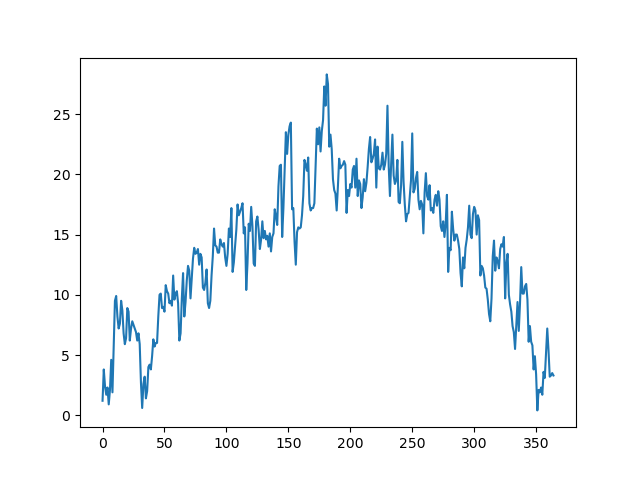# Line plot in Matplotlib

By Martin McBride, 2022-07-09
Tags: numeric python matplotlib line plot
Categories: matplotlib numpyWe have already looked briefly at line plots. A line plot is useful for presenting data that is quantitative (numerical) and where the data points have a natural order.

Now we will create a line graph using some real data - the daily UK temperatures from 2009. This is fairly similar to the first plot we made but using temperature data rather than artificial data.

We could just plot the temperature against the date as a series of points (that would be a scatter plot). But joining the points with a line emphasises the fact that the days have a certain sequence in time.

## The data

The file 2009-temp-daily.csv contains 365 values, representing the temperature for each day of the year 2009. This is described in the data article0.

## Plotting the data

The data provides the y values for the plot, but we also need the x values. We can simply use an incrementing count from 0 to 364 to represent the 365 days. So for example:

• Day 0 is the 1st of January.
• Day 7 is the 8th of January.
• Day 31 is the first of February, and so on.

Then we need to call the plot and show functions in the same way as we did before. Here is the full code:

import matplotlib.pyplot as plt
import csv

with open("2009-temp-daily.csv") as csv_file:
temperature = [x for x in csv_reader]

days = range(365)
plt.plot(days, temperature)
plt.show()


The code is here on github, in the file temperature-plot.py.

The first part of this code reads in the data. This is the same code we looked at in the data article, so we won't explain it again here. The code creates a list temperature containing the 365 daily values.

Here is how we create the x values (which we will store in a list called days):

days = range(365)


The easiest way to create the days list is to use the range function. This is the same function that you would often use in a for loop. range(365) creates a sequence that counts from 0 to 364, which is exactly what we want.

This is how we plot the line graph:

plt.plot(days, temperature)
plt.show()


The call to the plot function is similar to the previous example, except that we use days and temperature rather than x and y. The call to the show function is identical to the previous example.

## Final temperature graph

Here is the result:It is pretty much what you might expect, generally cold in the winter and warmish in the summer, with quite a lot of daily variation.

One thing to notice is that the axes have been automatically scaled to match the range of days and temperature. This is often quite a useful feature, although we will see later how to control the ranges manually if you ever need to.

## Formatting the line

We can change the appearance of the line using various formatting options provided by Matplotlib:

• The fmt parameter uses a string to specify basic colour and line style options. Use this for simple formatting.
• The color parameter sets the line colour, using named colours of RGB values.
• The linewidth parameter sets the width of the line.
• The linestyle parameter can create dashed lines of various types.

We can also add markers (a small circle or other shape that marks each data point on the line).

These options are covered in more detail in the article line and marker styles.